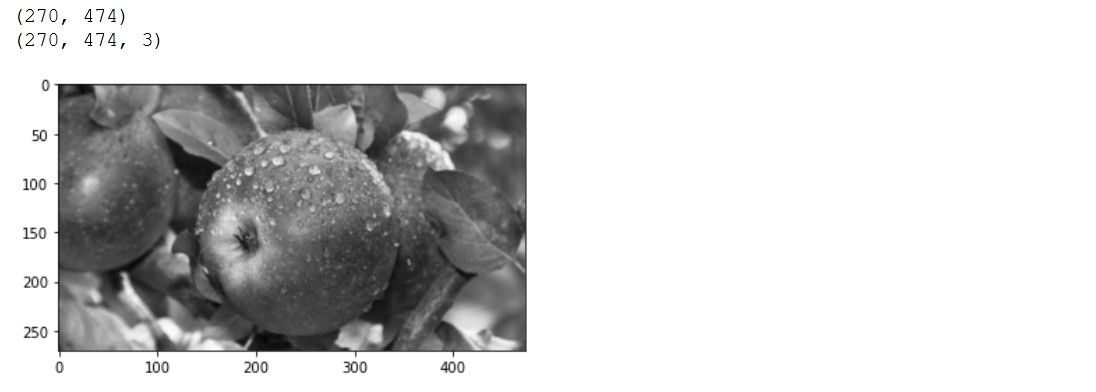OpenCV Create and Show the Image Correctly

Usually when we think of images we imagine three channel images.

Let’s create the image in Python using just matplotlib.pyplot and numpy packages. One great example would be to create a three channel gray image.

Create a gray image

#create gray image 270x474 with 3 channels
%matplotlib inline
import matplotlib.pyplot as plt
import numpy as np

img = np.empty([270,474, 3], dtype=np.uint8)
img.fill(128) # gray

plt.imshow(img)
plt.show()

print(img.shape)
print(type(img))

In this example we created a 3 channel image and filled it in gray.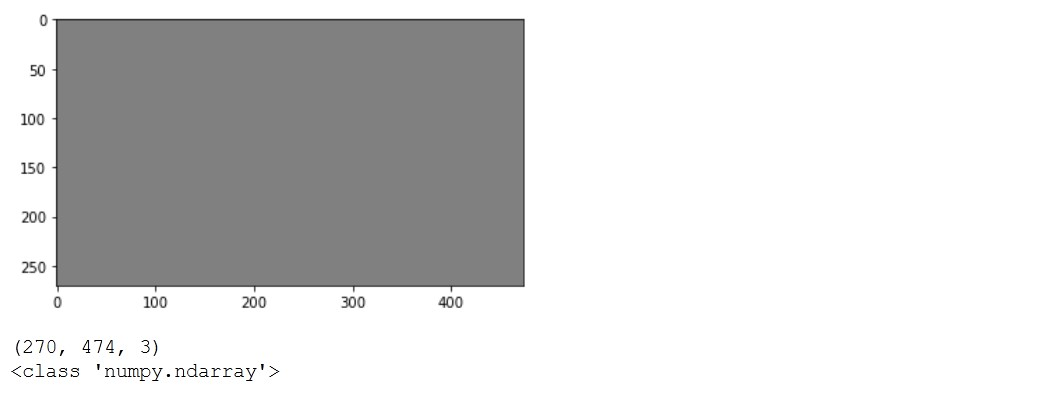Draw primitives

Lines and circles are basic primitives we can add to the image. We use OpenCV library for adding primitives because it works directly on numpy images.

import numpy as np
import cv2

img = np.zeros((270,474, 3), dtype = "uint8")

green = (0, 255, 0)
cv2.line(img, (0, 0), (474, 270), green)

red = (255, 0, 0)
R = 100
(centerX, centerY) = (img.shape // 2, img.shape // 2)
cv2.circle(img, (centerX, centerY), R , red)

plt.imshow(img)
plt.show()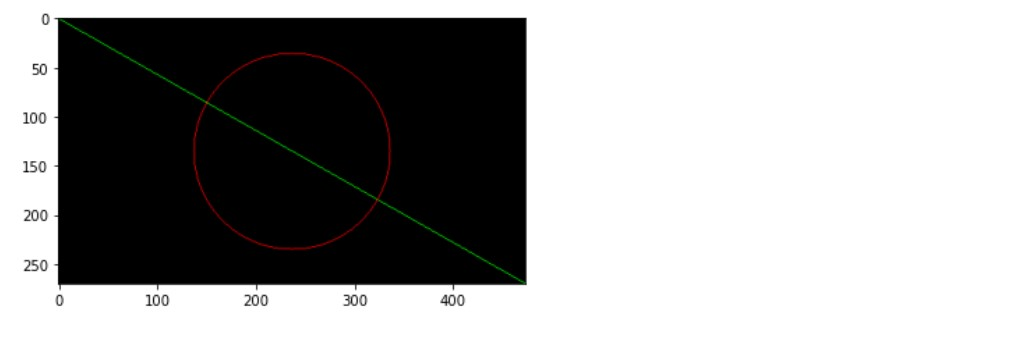We used matplotlib.pyplot to show the image.

Create a noisy image

Again we will create the image just using matplotlib.pyplot and numpy.

import matplotlib.pyplot as plt
import numpy as np
img = np.random.rand(270,474,3)
plt.imshow(img)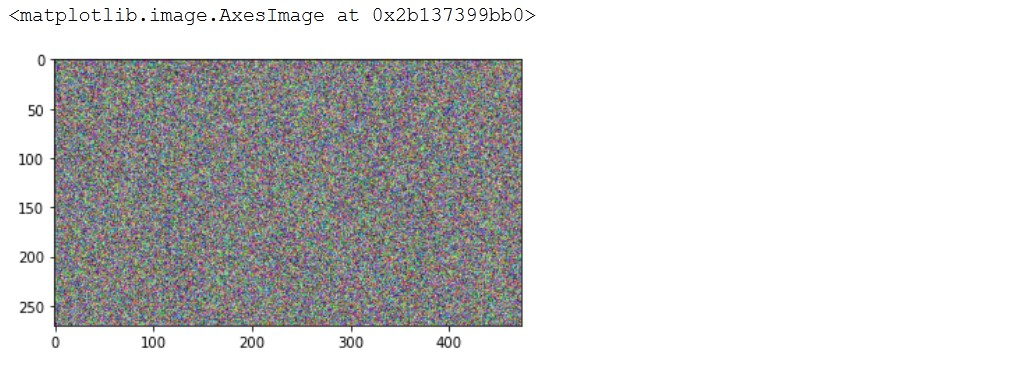Here we are using the matplotlib.pyplot method imshow again.

Next we will read the image from the URL and save it to the file system.

import requests
url = 'https://external-content.duckduckgo.com/iu/?u=https%3A%2F%2Ftse1.mm.bing.net%2Fth%3Fid%3DOIP.MBtx9vFOK2x5CM9Jjx0M5AHaEO%26pid%3DApi&f=1'
response = requests.get(url, allow_redirects=True) # nostream
with open('apple.jpg', 'wb') as handle:
handle.write(response.content)

# saved as C:\Users\dj\apple.jpg
print(handle)

Show image

Now we will just read the apple.jpg image using cv2 Python library.

%matplotlib inline
from matplotlib import pyplot as plt
import cv2
print(img.shape)
img=img[:,:,::-1]
_ = plt.imshow(img)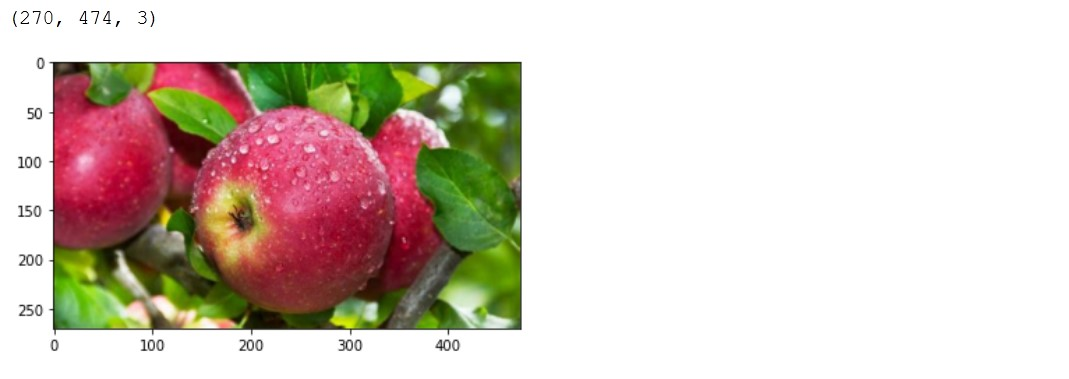Blue apple

If we would not convert the image with img=img[:,:,::-1] we would get the blue apple.

%matplotlib inline
from matplotlib import pyplot as plt
import cv2
_ = plt.imshow(img)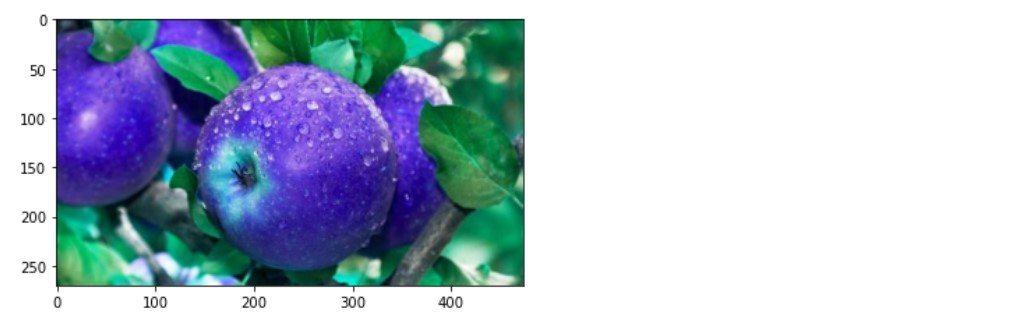Create a grayscale image from RGB

We can just use OpenCV cvtColor method to create single channel image and then if we combine three of these using OpenCV merge method we will get the gray apple.

img = cv2.cvtColor(img, cv2.COLOR_BGR2GRAY)
print(img.shape)
img = cv2.merge([img,img,img])
_ = plt.imshow(img)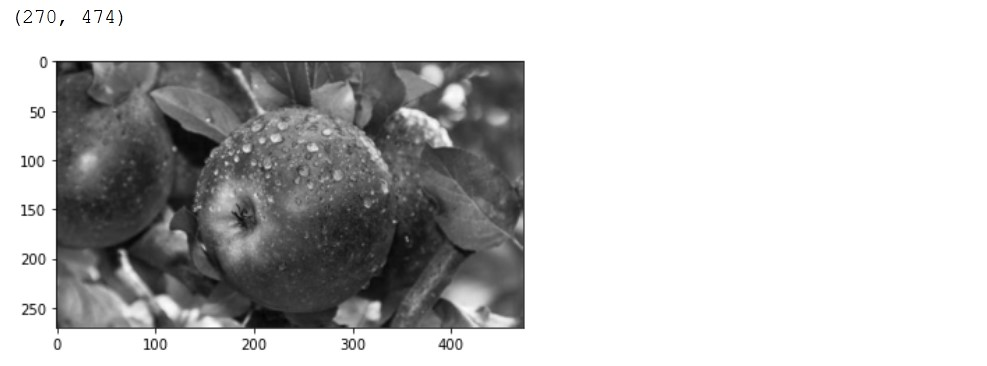Check out this gist for more details.

Show single channel image

%matplotlib inline
from matplotlib import pyplot as plt
import cv2
print(img.shape)
_ = plt.imshow(img)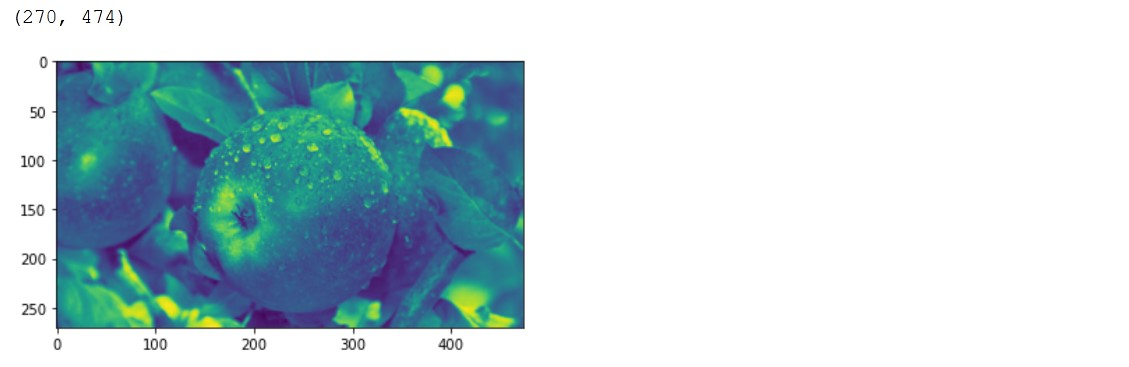But this does not look gray, because imshow method is using viridis cmap. Let’s use gray cmap.

_ = plt.imshow(img, cmap='gray') # default is viridis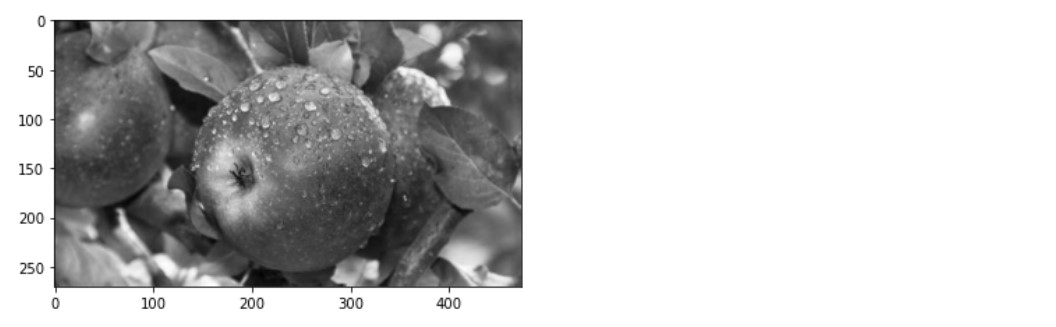Convert single channel image to RGB (three channels)

%matplotlib inline
from matplotlib import pyplot as plt
import cv2